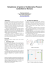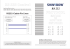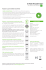# PDF - 1,07 MB - Bulletin of the Polish Academy of Sciences

## Transcription

PDF - 1,07 MB - Bulletin of the Polish Academy of Sciences
```BULLETIN OF THE POLISH ACADEMY OF SCIENCES
TECHNICAL SCIENCES
Vol. 56, No. 4, 2008
Stability regions of closed loop system with time delay inertial plant
of fractional order and fractional order PI controller
A. RUSZEWSKI∗
Faculty of Electrical Engineering, Białystok Technical University, 45D Wiejska St., 15-351 Białystok, Poland
Abstract. The paper presents the stability problem of control systems composed of a fractional-order PI controller and a inertial plant of
a fractional order with time delay. Simple and efficient computational method for determining stability regions in the controller and plant
parameters space is given. Knowledge of these regions permits tuning of the fractional-order PI controller. The method proposed is based
on the classical D-partition method.
Key words: PID controllers, fractional system, stability, delay, D-partition method.
1. Introduction
Proportional – Integral – Derivative (PID) controllers are
widely applied, because of their simple structure. PID-control
has been the subject of many publications (see for example [1–
4]). Many methods of tuning PID controllers for satisfactory behaviour have been proposed in the literature . These
methods are based on knowledge of the mathematical description of the process. The first order-plant with time delay is the
most frequently used model for tuning PID controller [1, 3].
The asymptotic stability of a closed-loop system is the
basic requirement. Several methods for determining the asymptotic stability regions in the controller parameter space
have been presented [2, 4–6]. These approaches are based
on the Pontryagin’s theory [2, 6] and generalization of the
Hermite-Biehler Theorem [4, 5]. An alternative approach to
the problem of stabilizing the first order-plant with time delay and multi-inertial plant with time delay was described
in [7–9]. Using the classical D-partition method, simple and
efficient computational methods of computing the asymptotic stability and D-stability region in the parameter space are
presented.
In recent years, considerable attention has been paid to
control systems whose processes and/or controllers are of
a fractional order (see for example [10–14]). The fractional
PID controllers, namely PIλ Dµ controllers, including an integrator of a λ order and a differentiator of a µ order have been
proposed in . Several design methods of tuning the PIλ Dµ
controllers for systems without time delay have been presented [13-16]. It has been shown that the PIλ Dµ controller which
has five degrees of freedom enhances the system control performance when used for control systems with IO (integer order) plants and FO (fractional order) plants. A computation
method of stabilizing fractional-order PIλ Dµ controllers for
fractional-order time delay systems was presented in .
In this paper a simple method of determining the stability
region in the parameter space of a inertial plant of a fractional
∗ e-mail:
order with time delay and a fractional-order PI controller is
given. Using this region, a very fast and simple way of calculating the stabilizing values of PIλ controllers is obtained.
Four cases for closed-loop control systems are analysed: 1) IO
controller with IO plant; 2) IO controller with FO plant; 3) FO
controller with IO plant and 4) FO controller with FO plant.
The integer order PI controller and a plant are special cases of
the fractional PI controller and a plant. The method proposed
is based on the D-partition method and an approach given
in [7, 18].
2. Problem formulation
Consider the feedback control system shown in Fig. 1 in which
the process to be controlled is described by an inertial plant
with time delay
Ke−sh
G(s) =
,
(1)
1 + sα T
where K, T , h are positive real numbers and the order α can
be integer (α = 1) or fractional with 0 < α < 1.
r
C (s)
u
G (s)
y
-
Fig. 1. Feedback control system
Let the controller C(s) be of the fractional PI with the
transfer function 
ki
C(s) = kp + λ ,
(2)
s
where kp and ki denote the proportional and integral gains
of the controller, and λ is the fractional order of the integrator (the order may assume positive real noninteger values).
Clearly, on selecting λ = 1, a classical PI controller can be
obtained.
[email protected]
329
A. Ruszewski
The characteristic function of the closed-loop system with
plant (1) and controller (2) is given by
λ
α
λ
w(s) = K(kp s + ki ) exp(−sh) + (1 + T s )s .
(3)
The closed-loop system in Fig. 1 is said to be boundedinput bounded-output stable if and only if all the zeros of the
characteristic function (3) have negative real parts. It is noted
that (3) is a quasi-polynomial which has an infinite number
of zeros. This makes the problem of analysing the stability
of the closed-loop system difficult. There is no general algebraic methods available in the literature for the stability test
of quasi-polynomials. In general, the stability test of a quasipolynomial is performed with a graphical method, e.g., the
Mikhajlov criterion. Algebraic methods are only known for
a particular class of quasi polynomials [6, 19, 20]. The next
problem of synthesis of the closed-loop system is how to
choose such a fractional order λ of the integrator that the
closed-loop system will be stable.
3. Main results
Multiplying quasi-polynomial (3) by exp(sh) we obtain a new
quasi-polynomial in the form
w(s) = K(kp sλ + ki ) + sλ (1 + T sα ) exp(sh),
(4)
which has exactly the same zeros as quasi-polynomial (3).
Substituting z = sh in quasi-polynomial (4) after transformations we obtain the quasi-polynomial
w(z) = Xz λ + Y + z λ (1 + pz α ) exp(z),
λ
(5)
α
pω λ+α sin
π
(λ + α) + ω + ω λ sin
λ+ω
2
2
π λ
+ Xω sin
λ = 0.
2
π
(9)
Finally, by solving the Eqs. (8) and (9) we obtain
π
π
i
−1 h α
X=
(λ
+
α)
+
ω
+
sin
λ
+
ω
,
pω
sin
2
2
sin π2 λ
(10)
i
h
1
π
pω λ+α sin
α + ω + ω λ sin (ωh) .
Y =
π
2
sin 2 λ
(11)
Equations (10) and (11) determine the complex zero
boundary in plane (X, Y ). The real zero boundary and the
complex zero boundary for ω > 0 decompose plane (X, Y )
into regions D(k). The stability region D(0) is chosen by
testing an arbitrary point from each region and checking the
stability of the quasi-polynomial (5) using the methods proposed in .
First, we analyse the asymptotic stability regions of quasipolynomial (5) for case 1 (IO controller with IO plant). This
was derived in . Figure 2 shows the asymptotic stability
regions of quasi-polynomial (5) for λ = 1, α = 1 and different values of p = T /h. Any point from these regions ensures
the stability of the control system considered. It is observed
from Fig. 2 that the increasing value of p results in larger
stability regions, because of the increase ranges of X = Kkp
and Y = Kki h.
where X = Kkp , Y = Kki h , p = T /h .
Using the D-partition method  the asymptotic stability region in the parameter plane (X, Y ) may be determined
and the parameters can be specified. The plane (X, Y ) is
decomposed by the so-called boundaries of D-partition into
finite number regions D(k). Any point in D(k) corresponds
to such values of X and Y that quasi-polynomial (5) has
exactly k zeros with positive real parts. The region D(0), if
it exists, is the stability region of quasi-polynomial (5). The
D-partition boundaries are curves on which each point corresponds to quasi-polynomial (5) having zeros on the imaginary
axis. It may be the real zero boundary or the complex zero
boundary. It is easy to see that quasi-polynomial (5) has zero
z = 0 if Y = 0 (the real zero boundary). The complex zero
boundary corresponds to the pure imaginary zeros of (5). We
obtain this boundary by solving the equation
w(jω) = X(jω)λ +Y +(jω)λ [1+p(jω)α ] exp(jω) = 0, (6)
which we get by substituting z = jω in quasi-polynomial (5)
and equating to 0. The term of j λ which is required for equation (6) can be expressed by
π π λ + j sin
λ .
(7)
j λ = cos
2
2
Using (7) the complex equation (6) can be rewritten as
a set of real equations in the form
π
π
pω λ+α cos
(λ + α) + ω + ω λ cos
λ+ω
2
2
π (8)
λ
+ Xω cos
λ + Y = 0,
2
330
Fig. 2. Stability regions for quasi-polynomial (5) for λ = 1, α = 1
Next we consider the case with IO controller and FO plant.
Figure 3a shows the stability regions of quasi-polynomial (5)
for λ = 1 and p = 4 and different values of the fractional order of plant α ∈ (0, 1). We can see that the stability regions
in this case are larger than in the previous one. The use of the
fractional order of a plant causes an increase in the stability
regions. The increasing value of p entails increasing stability
regions (Fig. 3b).
Bull. Pol. Ac.: Tech. 56(4) 2008
Stability regions of closed loop system with time delay inertial plant of fractional order...
Fig. 3. Stability regions for quasi-polynomial (5) for λ = 1; a) p = 4,
b) α = 0.5
Fig. 5. Stability regions for quasi-polynomial (5) for p = 4;
a) λ = 0.5, b) α = 0.5
The stability regions for the case with FO controller and
IO plant is shown in Fig. 4. The figure shows that for λ < 1
the stability regions are larger than for λ = 1. An increase
in the value of λ to over one initially results in an increase
in the stability region after which it begins to decrease. The
value of λ at which the stability region disappears is λ = 2.
The result of an increase in the value of p is the same as in
case 1 (Fig. 4b).
To sum up, we can see that increasing the value of p
causes an increase in the stability regions with IO plant and
with FO plant. The fractional order of the controller entails
increasing stability regions both with IO plant and FO plant
when λ < 1. An excessive value of λ results in the disappearance of the stability region. The value of λ at which the
stability region disappears is λ = 2.
4. Example
Consider the feedback control system shown in Fig. 1 in which
the process to be controlled is described by transfer function
(1) where K = 1, h = 0.5, T = 2.
First we analyse the closed-loop system with IO controller
and IO plant (case 1). The stability regions are shown in
Fig. 2. In the example we have p = T /h = 4, thus the stability region is limited by the line Y = 0 and the curve
corresponds to p = 4. Choosing an arbitrary point from this
region, e.g. X = 2, Y = 1, we obtain Kkp = 2, Kki hλ = 1.
By computation based on the above expressions the following
stabilizing controller parameters kp = 2, ki = 2 are obtained.
In a similar manner we can determine the transfer function of
the controller (2) in others cases:
Fig. 4. Stability regions for quasi-polynomial (5) for α = 1; a) p = 4,
b) λ = 0.5
Figure 5 shows the stability regions of quasi-polynomial
(5) for the case with FO controller and FO plant. We can
see that the stability regions in this case are larger than in all
the previous ones. Like the case with FO controller and IO
plant, an excessive increase in the value of λ results in the
disappearance of the stability region.
Bull. Pol. Ac.: Tech. 56(4) 2008
2) IO controller with FO plant λ = 1, α = 0.5, X = 2,
8
Y = 4, C(s) = 2 + ,
s
3) FO controller with IO plant λ = 0.5, α = 1, X = 2,
2.828
Y = 2, C(s) = 2 + 0.5 ,
s
4) FO controller with FO plant λ = 0.5, α = 0.5, X = 2,
5.657
Y = 4, C(s) = 2 + 0.5 .
s
It is noted that point X = 2, Y = 4 lies outside the stability region for the case with IO controller and IO plant (Fig. 2).
In case 3 (FO controller, IO plant) this point also lies outside
the stability region for the value λ = 0.5. In order to ensure
331
A. Ruszewski
that this point lies in the stability region we have to decrease
the value of λ. That point lies in the stability region for the
case with FO controller and FO plant for λ = 0.5, α = 0.5
and for the case with IO controller and FO plant for α = 0.5.
5. Concluding remarks
In this paper, the stability problem of control systems composed of a fractional-order PI controller and a inertial plant
of fractional order with time delay is examined. Four cases of
closed-loop control systems have been analysed: 1) IO controller with IO plant; 2) IO controller with FO plant; 3) FO
controller with IO plant and 4) FO controller with FO plant.
The integer order PI controller and plant are special cases of
the fractional PI controller and plant. On the basis of the Dpartition method, analytical forms expressing the D-partition
boundaries of stability regions in the parameter space were
determined. Knowledge of stability regions permits tuning of
the fractional PI type controller. This study shows that a PIλ
controller which has three degrees of freedom increases the
stability regions for λ < 1 when used for control systems with
IO and FO process models with time delay.
The studies can be extended for the problem of stabilization using a fractional-order PID controller.
Acknowledgements. This work was supported by Ministry
of Science and Higher Education in Poland under work
No N N514 1939 33.
REFERENCES
 K.J. Aström and T. Hägglund, PID Controllers: Theory, Design, and Tuning, NC: Instrument Society of America, USA,
1995.
 H. Górecki, Analysis and Synthesis of Time Delay Systems,
WNT, Warsaw, 1971, (in Polish).
 A. O’Dwyer, PI and PID Controller Tuning Rules, Imperial
College Press/Word Scientific, London, 2003.
 G.J. Silva, A. Datta, and S.P. Bhattacharyya, PID Controllers
for Time-Delay Systems, Birkhauser, Boston, 2005.
 A. Datta, M.-T Ho, and S.P. Bhattacharyya, Structure and Synthesis of PID Controllers, Springer-Verlag, London, 2000.
 J.E. Marshal, H. Górecki, K. Walton, and A. Korytowski, Time
Delay Systems – Stability and Performance Criteria with Application, Ellis Horwood, Chichester, 1992.
 M. Busłowicz and A. Ruszewski, “Stabilization of first order
systems with delay using the PI controllers”, Proc. XIV National Conference of Automatics 1, 89–94 (2002), (in Polish).
332
 A. Ruszewski, “Stability regions of control systems with multiinertial plant with delay in the parameter space”, Proc. XV National Conference of Automatics 1, 189–192 (2005), (in Polish).
 A. Ruszewski, Parametric Synthesis of Controllers for Particular Plants with Uncertain Parameters, PhD Dissertation,
Faculty of Electrical Engineering, Białystok, 2008, (in Polish).
 M. Busłowicz, “Stability of linear continuous-time fractional
order systems with delays of the retarded type”, Bull. Pol. Ac.:
Tech. 56 (4), (2008).
 D. Matignon, “Stability properties for generalized fractional
differential systems”, Proc. ESAIM 145–158 (1998).
 P. Ostalczyk, Outline of Fractional Order Integral-differential
Calculus. Theory and Application in Automatics, Publishing
Department of Technical University of Łódź, Łódź, 2008, (in
Polish).
 I. Podlubny, “Fractional-order systems and PIλ Dµ – controllers”, IEEE Trans. on Automatic Control 44, 208–214
(1999).
 C. Zhao, D. Xue, and Y.Q. Chen, “A fractional order PID tuning algorithm for a class of fractional order plants”, Proc. IEEE
Int. Conf. on Mechatronics & Automation 216–221 (2005).
 Y.Q. Chen, H. Dou, B.M. Vinagre, and C.A. Monje, “A robust
tuning method for fractional order PI controllers”, The Second
IFAC Symposium on Fractional Derivatives and Applications
FDA06, CD-ROM (2006).
 C.A. Monje, B.M. Vinagre, Y.Q. Chen, V. Feliu, P. Lanusse,
and J. Sabatier, “Proposals for fractional PIλ Dµ tuning”, The
First IFAC Symposium on Fractional Differentiation and its
Applications 38, 369–381 (2004).
 S.E. Hamamci, “An algorithm for stabilization of fractionalorder time delay systems using fractional-order PID controllers”, IEEE Trans. on Automatic Control 52, 1964–1969
(2007).
 A. Ruszewski, “Stabilization of fractional-order Strejc’s
process model with time delay using fractional-order PI controller”, in Recent Advances in Control and Automation, pp.
103–113, eds: K. Malinowski and L. Rutkowski, Academic
Publishing House Exit, Warsaw, 2008.
 H. Górecki, S. Fuksa, P. Grabowski, and A. Korytowski, Analysis and Synthesis of Time Delay Systems, PWN-J. Wiley,
Warsaw-Chichester, 1989.
 H. Górecki and A. Korytowski, Advances in Optimization and
Stability Analysis of Dynamical Systems, Publishing Department of University of Mining and Metallurgy, Kraków, 1993.
 M. Busłowicz, “Frequency domain method for stability analysis of linear continuous-time fractional systems”, in Recent
Advances in Control and Automation, pp. 83–92, eds.: K. Malinowski and L. Rutkowski, Academic Publishing House Exit,
Warsaw, 2008.
Bull. Pol. Ac.: Tech. 56(4) 2008
```

### portable usb dj mixer + scratch controll er + dj software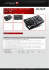### PeopleSoft Finance - Pain Points/Talking Points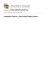### Mega Plexxus Instruction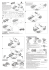### X-Zec 5-W Schenck/Horiba dynamometers controller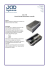### IMSce™ for V5/V6 - IMS Software, Inc.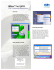### argos PS3 Pro Elite User Guide.indd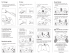### Zone Control Product Bulletin - Mitsubishi Electric Australia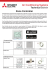### Simpletones: A System of Collaborative Physical Controllers for